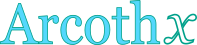## Inverse hyperbolic cotangent calculator

This tool evaluates the inverse hyperbolic cotangent of a number: arcoth(x). Enter the argument x below.

 x = Result: arcoth(x) =## Definitions

### General

The inverse hyperbolic cotangent function, in modern notation written as arcoth(x) or arccoth(x) or coth-1x, gives the value t (hyperbolic angle), so that:

The inverse hyperbolic cotangent function accepts arguments in real open intervals (-∞,-1) and (1,∞), because for all non-zero real x. Since the hyperbolic cotangent is defined through the natural exponential function , its inverse can be defined through the natural logarithm function, using the following formula, for real x, with |x|>1:

### Properties

The derivative of the inverse hyperbolic cotangent function is:

The integral of the inverse hyperbolic cotangent function is given by: